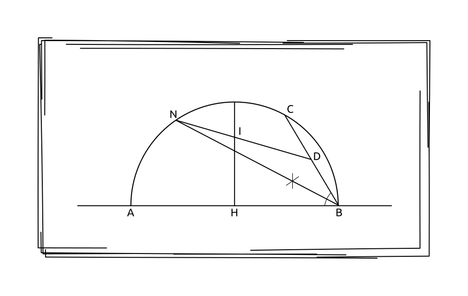# Problem Number Nine and Three Quartermaruf_0011SUST Inter University Pro...
Limits 1s, 512 MB

Harry is going to start his school at Hogwarts this year. In order to reach Hogwarts, he needs to catch the “Hogwarts Express” and that too from platform number nine and three quarter of the King’s Cross railway station. But, he doesn’t know how far he has to go to reach that peculiar platform.

Assume, King’s Cross is a railway station in a half-circular shape. Harry is standing on the midpoint of diameter $AB$, which is $H$. There is a chord $BC$. The bisector $BN$ of the angle $∠ABC$ intersects King’s Cross at $N$. The midpoint of $BC$ is $D$. $DN$ intersects the perpendicular bisector of the chord $AB$ at $I$, exactly where the platform nine and three quarter is located.Harry needs to reach $I$ from $H$. He only knows the radius of King’s Cross railway station $r$, and the value of the angle $∠ABC$. Help Harry calculate the length of $HI$.

## Input

Input starts with an integer $T$ ($T ≤ 100$), denotes the number of test cases.

Then T lines follow. Each line contains two integers $r$ ($1 ≤ r ≤ 100$), denoting the radius of King’s Cross and $x$ ($5 ≤ x ≤ 85$), denoting the value of angle $∠ABC$, in degrees.

## Output

For each test case, print the length of $HI$. Errors less than $10^{-6}$ will be ignored.

## Sample

InputOutput
2
10 45
50 30

5.857864
22.400924GeometryuDebug

### Submit